# Continuous function

Continuous function

In mathematics, a continuous function is a function for which, intuitively, small changes in the input result in small changes in the output. Otherwise, a function is said to be "discontinuous." A continuous function with a continuous inverse function is called "bicontinuous."

Continuity of functions is one of the core concepts of topology, which is treated in full generality below. The introductory portion of this article focuses on the special case where the inputs and outputs of functions are real numbers. In addition, this article discusses the definition for the more general case of functions between two metric spaces. In order theory, especially in domain theory, one considers a notion of continuity known as Scott continuity. Other forms of continuity do exist but they are not discussed in this article.

As an example, consider the function h(t), which describes the height of a growing flower at time t. This function is continuous. In fact, a dictum of classical physics states that in nature everything is continuous. By contrast, if M(t) denotes the amount of money in a bank account at time t, then the function jumps whenever money is deposited or withdrawn, so the function M(t) is discontinuous.

## Real-valued continuous functions

### Definition

A function from the set of real numbers to the real numbers can be represented by a graph in the Cartesian plane; the function is continuous if, roughly speaking, the graph is a single unbroken curve with no "holes" or "jumps".

There are several ways to make this intuition mathematically rigorous. These definitions are equivalent to one another, so the most convenient definition can be used to determine whether a given function is continuous or not. In the definitions below,$f: I \rightarrow \mathbf R.$

is a function defined on a subset I of the set R of real numbers. This subset I is referred to as the domain of f. Possible choices include I=R, the whole set of real numbers, an open interval$I = (a, b) = \{x \in \mathbf R | a < x < b \},$

or a closed interval$I = [a, b] = \{x \in \mathbf R | a \leq x \leq b \}.$

Here, a and b are real numbers.

#### Definition in terms of limits of functions

The function f is continuous at some point c of its domain if the limit of f(x) as x approaches c through domain of f exists and is equal to f(c). In mathematical notation, this is written as$\lim_{x \to c}{f(x)} = f(c).$

In detail this means three conditions: first, f has to be defined at c. Second, the limit on the left hand side of that equation has to exist. Third, the value of this limit must equal f(c).

The function f is said to be continuous if it is continuous at every point of its domain. If the point c in the domain of f is not a limit point of the domain, then this condition is vacuously true, since x cannot approach c through values not equal c. Thus, for example, every function whose domain is the set of all integers is continuous.

#### Definition in terms of limits of sequences

One can instead require that for any sequence$(x_n)_{n\in\mathbb{N}}$ of points in the domain which converges to c, the corresponding sequence$\left(f(x_n)\right)_{n\in \mathbb{N}}$ converges to f(c). In mathematical notation,$\forall (x_n)_{n\in\mathbb{N}} \subset I:\lim_{n\to\infty} x_n=c \implies \lim_{n\to\infty} f(x_n)=f(c)\,.$

#### Weierstrass definition (epsilon-delta) of continuous functionsIllustration of the ε-δ-definition: for ε=0.5, the value δ=0.5 satisfies the condition of the definition.

Explicitly including the definition of the limit of a function, we obtain a self-contained definition: Given a function f as above and an element c of the domain I, ƒ is said to be continuous at the point c if the following holds: For any number ε > 0, however small, there exists some number δ > 0 such that for all x in the domain of ƒ with c − δ < x < c + δ, the value of ƒ(x) satisfies$f(c) - \varepsilon < f(x) < f(c) + \varepsilon.\,$

Alternatively written, continuity of ƒ : I → D at c ∈ I means that for every ε > 0 there exists a δ > 0 such that for all x ∈ I,:$| x - c | < \delta \Rightarrow | f(x) - f(c) | < \varepsilon. \,$

A form of this epsilon-delta definition of continuity was first given by Bernard Bolzano in 1817. Preliminary forms of a related definition of the limit were given by Cauchy, though the formal definition and the distinction between pointwise continuity and uniform continuity were first given by Karl Weierstrass.

More intuitively, we can say that if we want to get all the ƒ(x) values to stay in some small neighborhood around ƒ(c), we simply need to choose a small enough neighborhood for the x values around c, and we can do that no matter how small the ƒ(x) neighborhood is; ƒ is then continuous at c.

In modern terms, this is generalized by the definition of continuity of a function with respect to a basis for the topology, here the metric topology.

#### Definition using oscillation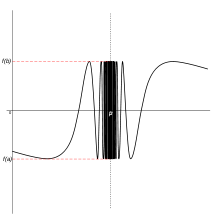The failure of a function to be continuous at a point is quantified by its oscillation.

Continuity can also be defined in terms of oscillation: a function ƒ is continuous at a point x0 if and only if the oscillation is zero; in symbols, ωf(x0) = 0. A benefit of this definition is that it quantifies discontinuity: the oscillation gives how much the function is discontinuous at a point.

This definition is useful in descriptive set theory to study the set of discontinuities and continuous points – the continuous points are the intersection of the sets where the oscillation is less than ε (hence a Gδ set) – and gives a very quick proof of one direction of the Lebesgue integrability condition.

The oscillation is equivalent to the ε-δ definition by a simple re-arrangement, and by using a limit (lim sup, lim inf) to define oscillation: if (at a given point) for a given ε0 there is no δ that satisfies the ε-δ definition, then the oscillation is at least ε0, and conversely if for every ε there is a desired δ, the oscillation is 0. The oscillation definition can be naturally generalized to maps from a topological space to a metric space.

#### Definition using the hyperreals

Cauchy defined continuity of a function in the following intuitive terms: an infinitesimal change in the independent variable corresponds to an infinitesimal change of the dependent variable (see Cours d'analyse, page 34). Non-standard analysis is a way of making this mathematically rigorous. The real line is augmented by the addition of infinite and infinitesimal numbers to form the hyperreal numbers. In nonstandard analysis, continuity can be defined as follows.

A function ƒ from the reals to the reals is continuous if its natural extension to the hyperreals has the property that for real x and infinitesimal dx, ƒ(x+dx) − ƒ(x) is infinitesimal.

In other words, an infinitesimal increment of the independent variable corresponds to an infinitesimal change of the dependent variable, giving a modern expression to Augustin-Louis Cauchy's definition of continuity.

### Examples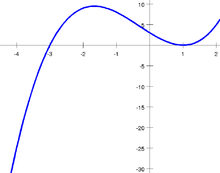The graph of a cubic function has no jumps or holes. The function is continuous.

All polynomial functions, such as$f(x) = x^3 + x^2 - 5x + 11\,$

(pictured) are continuous. This is a consequence of the fact that, given two continuous functions$f, g: I \rightarrow \mathbf R$

defined on the same domain I, then the sum f + g, and the product fg of the two functions are continuous (on the same domain I). Moreover, the function$\frac f g : \{ x \in I| g(x) \neq 0 \} \rightarrow \mathbf R, x \mapsto \frac{f(x)}{g(x)}$

is continuous. (The points where g(x) is zero have to be discarded for f/g to be defined.) For example, the function (pictured)$f(x) = \frac {2x-1} {x+2}$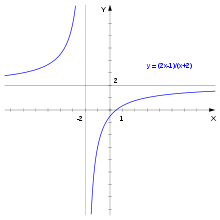The graph of a rational function. The function is not defined for x=−2. The vertical and horizontal lines are asymptotes.

is defined for all real numbers x ≠ −2 and is continuous at every such point. The question of continuity at x = −2 does not arise, since x = −2 is not in the domain of f. There is no continuous function F: RR that agrees with f(x) for all x ≠ −2. The function g(x) = (sin x)/x, defined for all x≠0 is continuous at these points. However, this function can be extended to a continuous function on all real numbers, namely$G(x) = \begin{cases} \frac {\sin (x)}x & \mbox{ if }x \ne 0\\ 1 & \mbox{ if }x = 0, \end{cases}$

since the limit of g(x), when x approaches 0, is 1. Therefore, the point x=0 is called a removable singularity of g.

Given two continuous functions$f: I \rightarrow J (\subset \mathbf R), g : J \rightarrow \mathbf R,$

the composition$g \circ f : I \rightarrow \mathbf R, x \mapsto g(f(x))$

is continuous.

### Non-examples

An example of a discontinuous function is the function f defined by f(x) = 1 if x > 0, f(x) = 0 if x ≤ 0. Pick for instance ε = 12. There is no δ-neighborhood around x = 0 that will force all the f(x) values to be within ε of f(0). Intuitively we can think of this type of discontinuity as a sudden jump in function values. Similarly, the signum or sign function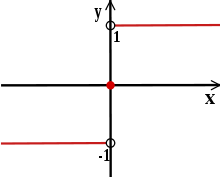Plot of the signum function. The hollow dots indicate that sgn(x) is 1 for all x>0 and −1 for all x<0.$\sgn(x) = \begin{cases} 1 & \mbox{ if }x > 0\\ 0 & \mbox{ if }x = 0\\ -1 & \mbox{ if }x < 0\\ \end{cases}$

is discontinuous at x=0 but continuous everywhere else. Yet another example: the function$f(x)=\begin{cases} \sin(\frac{1}{x^2})\mbox{ if }x \ne 0\\ 0\mbox{ if }x = 0 \end{cases}$

is continuous everywhere apart from x = 0.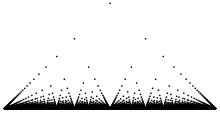Plot of Thomae's function for the domain −1 ≤ x ≤ 1.$f(x)=\begin{cases} 1 \mbox{ if }x=0\\ \frac{1}{q}\mbox{ if }x=\frac{p}{q}\mbox{ is a rational number}\\ 0\mbox{ if }x\mbox{ is irrational}. \end{cases}$

is continuous at all irrational numbers and discontinuous at all rational numbers. In a similar vein, Dirichlet's function$D(x)=\begin{cases} 0\mbox{ if }x \in \mathbb{R} \setminus \mathbb{Q}\\ 1\mbox{ if }x \in \mathbb{Q} \end{cases}$

is nowhere continuous.

### Properties

#### Intermediate value theorem

The intermediate value theorem is an existence theorem, based on the real number property of completeness, and states:

If the real-valued function f is continuous on the closed interval [ab] and k is some number between f(a) and f(b), then there is some number c in [ab] such that f(c) = k.

For example, if a child grows from 1 m to 1.5 m between the ages of two and six years, then, at some time between two and six years of age, the child's height must have been 1.25 m.

As a consequence, if f is continuous on [ab] and f(a) and f(b) differ in sign, then, at some point c in [ab], f(c) must equal zero.

#### Extreme value theorem

The extreme value theorem states that if a function f is defined on a closed interval [a,b] (or any closed and bounded set) and is continuous there, then the function attains its maximum, i.e. there exists c ∈ [a,b] with f(c) ≥ f(x) for all x ∈ [a,b]. The same is true of the minimum of f. These statements are not, in general, true if the function is defined on an open interval (a,b) (or any set that is not both closed and bounded), as, for example, the continuous function f(x) = 1/x, defined on the open interval (0,1), does not attain a maximum, being unbounded above.

#### Relation to differentiability and integrability$f: (a, b) \rightarrow \mathbf R$

is continuous, as can be shown. The converse does not hold: for example, the absolute value function$f(x)=|x| = \begin{cases} x \mbox{ if }x \geq 0\\ -x\mbox{ if }x < 0 \end{cases}$

is everywhere continuous. However, it is not differentiable at x = 0 (but is so everywhere else). Weierstrass's function is everywhere continuous but nowhere differentiable.

The derivative f'(x) of a differentiable function f(x) need not be continuous. If f'(x) is continuous, f(x) is said to be continuously differentiable. The set of such functions is denoted C1((a, b)). More generally, the set of functions$f: \Omega \rightarrow \mathbf R$

(from an open interval (or open subset of R) Ω to the reals) such that f is n times differentiable and such that the n-th derivative of f is continuous is denoted Cn(Ω). See differentiability class. In the field of computer graphics, these three levels are sometimes called G0 (continuity of position), G1 (continuity of tangency), and G2 (continuity of curvature).

Every continuous function$f: [a, b] \rightarrow \mathbf R$

is integrable (for example in the sense of the Riemann integral). The converse does not hold, as the (integrable, but discontinuous) sign function shows.

#### Pointwise and uniform limits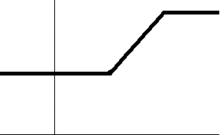A sequence of continuous functions fn(x) whose (pointwise) limit function f(x) is discontinuous. The convergence is not uniform.

Given a sequence$f_1, f_2, \dots : I \rightarrow \mathbf R$

of functions such that the limit$f(x) := \lim_{n \rightarrow \infty} f_n(x)$

exists for all x in I, the resulting function f(x) is referred to as the pointwise limit of the sequence of functions (fn)nN. The pointwise limit function need not be continuous, even if all functions fn are continuous, as the animation at the right shows. However, f is continuous when the sequence converges uniformly, by the uniform convergence theorem. This theorem can be used to show that the exponential functions, logarithms, square root function, trigonometric functions are continuous.

### Directional and semi-continuity

Discontinuous functions may be discontinuous in a restricted way, giving rise to the concept of directional continuity (or right and left continuous functions) and semi-continuity. Roughly speaking, a function is right-continuous if no jump occurs when the limit point is approached from the right. More formally, ƒ is said to be right-continuous at the point c if the following holds: For any number ε > 0 however small, there exists some number δ > 0 such that for all x in the domain with c < x < c + δ, the value of ƒ(x) will satisfy$|f(x) - f(c)| < \varepsilon.\,$

This is the same condition as for continuous functions, except that it is required to hold for x strictly larger than c only. Requiring it instead for all x with cδ < x < c yields the notion of left-continuous functions. A function is continuous if and only if it is both right-continuous and left-continuous.

A function f is upper semi-continuous if, roughly, any jumps that might occur only go up, but not down. That is, for any ε > 0, there exists some number δ > 0 such that for all x in the domain with |x − c| < δ, the value of ƒ(x) satisfies$f(x) \geq f(c) - \epsilon.$

## Continuous functions between metric spaces

The concept of continuous real-valued functions can be generalized to functions between metric spaces. A metric space is a set X equipped with a function (called metric) dX, that can be thought of as a measurement of the distance of any two elements in X. Formally, the metric is a function$d_X : X \times X \rightarrow \mathbf R$

that satisfies a number of requirements, notably the triangle inequality. Given two metric spaces (X, dX) and (Y, dY) and a function$f: X \rightarrow Y$

then f is continuous at the point c in X (with respect to the given metrics) if for any positive real number ε, there exists a positive real number δ such that all x in X satisfying dX(x, c) < δ will also satisfy dY(f(x), f(c)) < ε. As in the case of real functions above, this is equivalent to the condition that for every sequence (xn) in X with limit lim xn = c, we have lim f(xn) = f(c). The latter condition can be weakened as follows: f is continuous at the point c if and only if for every convergent sequence (xn) in X with limit c, the sequence (f(xn)) is a Cauchy sequence, and c is in the domain of f.

The set of points at which a function between metric spaces is continuous is a Gδ set – this follows from the ε-δ definition of continuity.

This notion of continuity is applied, for example, in functional analysis. A key statement in this area says that a linear operator$T: V \rightarrow W$

between normed vector spaces V and W (which are vector spaces equipped with a compatible norm, denoted ||x||) is continuous if and only if it is bounded, that is, there is a constant K such that$\|T(x)\| \leq K \|x\|$

for all x in V.

### Uniform, Hölder and Lipschitz continuity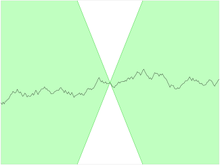For a Lipschitz continuous function, there is a double cone (shown in white) whose vertex can be translated along the graph, so that the graph always remains entirely outside the cone.

The concept of continuity for functions between metric spaces can be strengthened in various ways by limiting the way δ depends on ε and c in the definition above. Intuitively, a function f as above is uniformly continuous if the δ does not depend on the point c. More precisely, it is required that for every real number ε > 0 there exists δ > 0 such that for every cb ∈ X with dX(bc) < δ, we have that dY(f(b), f(c)) < ε. Thus, any uniformly continuous function is continuous. The converse does not hold in general, but holds when the domain space X is compact. Uniformly continuous maps can be defined in the more general situation of uniform spaces.

A function is Hölder continuous with exponent α (a real number) if there is a constant K such that for all b and c in X, the inequality$d_Y (f(b), f(c)) \leq K \cdot (d_X (b, c))^\alpha$

holds. Any Hölder continuous function is uniformly continuous. The particular case α = 1 is referred to as Lipschitz continuity. That is, a function is Lipschitz continuous if there is a constant K such that the inequality$d_Y (f(b), f(c)) \leq K \cdot d_X (b, c)$

holds any b, c in X. The Lipschitz condition occurs, for example, in the Picard–Lindelöf theorem concerning the solutions of ordinary differential equations.

## Continuous functions between topological spaces

Yet another, more abstract notion of continuity is continuity of functions between topological spaces. A topological space is a set X together with a set of subsets of X. These subsets are called open subsets. Intuitively, points belonging to some open subset are close to one another; however there is no formal notion of distance, as in the case of metric spaces. The set of open subsets needs to satisfy a few requirements in order to qualify for a topological space. If they are satisfied, this set of subsets is called a topology on the set X.

A function

f : XY

between two topological spaces X and Y is continuous if for every open set VY, the inverse image$f^{-1}(V) = \{x \in X \; | \; f(x) \in V \}$

is an open subset of X. That is, f is a function between the sets X and Y (not on the elements of the topology TX), but the continuity of f depend on the topologies used on X and Y.

This is equivalent to the condition that the preimages of the closed sets (which are the complements of the open subsets) in Y are closed in X.

An extreme example: if a set X is given the discrete topology (in which every subset is open), all functions$f: X \rightarrow T$

to any topological space T are continuous. On the other hand, if X is equipped with the indiscrete topology (in which the only open subsets are the empty set and X) and the space T set is at least T0, then the only continuous functions are the constant functions. Conversely, any function whose range is indiscrete is continuous.

### Alternative definitions

Several equivalent definitions for a topological structure exist and thus there are several equivalent ways to define a continuous function.

#### Neighborhood definition

Definitions based on preimages are often difficult to use directly. The following criterion expresses continuity in terms of neighborhoods: f is continuous at some point x ∈ X if and only if for any neighborhood V of f(x), there is a neighborhood U of x such that f(U) ⊆ V. Intuitively, continuity means no matter how "small" V becomes, there is always a U containing x that maps inside V.

If X and Y are metric spaces, it is equivalent to consider the neighborhood system of open balls centered at x and f(x) instead of all neighborhoods. This gives back the above δ-ε definition of continuity in the context of metric spaces. However, in general topological spaces, there is no notion of nearness or distance.

Note, however, that if the target space is Hausdorff, it is still true that f is continuous at a if and only if the limit of f as x approaches a is f(a). At an isolated point, every function is continuous.

#### Sequences and nets

In several contexts, the topology of a space is conveniently specified in terms of limit points. In many instances, this is accomplished by specifying when a point is the limit of a sequence, but for some spaces that are too large in some sense, one specifies also when a point is the limit of more general sets of points indexed by a directed set, known as nets. A function is continuous only if it takes limits of sequences to limits of sequences. In the former case, preservation of limits is also sufficient; in the latter, a function may preserve all limits of sequences yet still fail to be continuous, and preservation of nets is a necessary and sufficient condition.

In detail, a function f : XY is sequentially continuous if whenever a sequence (xn) in X converges to a limit x, the sequence (f(xn)) converges to f(x). Thus sequentially continuous functions "preserve sequential limits". Every continuous function is sequentially continuous. If X is a first-countable space and countable choice holds, then the converse also holds: any function preserving sequential limits is continuous. In particular, if X is a metric space, sequential continuity and continuity are equivalent. For non first-countable spaces, sequential continuity might be strictly weaker than continuity. (The spaces for which the two properties are equivalent are called sequential spaces.) This motivates the consideration of nets instead of sequences in general topological spaces. Continuous functions preserve limits of nets, and in fact this property characterizes continuous functions.

#### Closure operator definition

Instead of specifying the open subsets of a topological space, the topology can also be determined by closure operators (denoted cl) which assigns to any subset AX its closure or interior operators (denoted int), which assigns to any subset A of X its interior. In these terms, a function$f:(X,\mathrm{cl}) \to (X' ,\mathrm{cl}')\,$

between topological spaces is continuous in the sense above if and only if for all subsets A of X$f(\mathrm{cl}(A)) \subseteq \mathrm{cl}'(f(A)).$

That is to say, given any element x of X that is in the closure of any subset A, f(x) belongs to the closure of f(A). This is equivalent to the requirement that for all subsets A' of X'$f^{-1}(\mathrm{cl}'(A)) \supseteq \mathrm{cl}(f^{-1}(A)).$

Moreover,$f:(X,\mathrm{int}) \to (X' ,\mathrm{int}') \,$

is continuous if and only if$f^{-1}(\mathrm{int}'(A)) \subseteq \mathrm{int}(f^{-1}(A)).$

for any subset A of X.

### Properties

If f : XY and g : YZ are continuous, then so is the composition gf : XZ. If f : XY is continuous and

• X is compact, then f(X) is compact.
• X is connected, then f(X) is connected.
• X is path-connected, then f(X) is path-connected.
• X is Lindelöf, then f(X) is Lindelöf.
• X is separable, then f(X) is separable.

The possible topologies on a fixed set X are partially ordered: a topology τ1 is said to be coarser than another topology τ2 (notation: τ1 ⊆ τ2) if every open subset with respect to τ1 is also open with respect to τ2. Then, the identity map

idX : (X, τ2) → (X, τ1)

is continuous if and only if τ1 ⊆ τ2 (see also comparison of topologies). More generally, a continuous function$(X, \tau_X) \rightarrow (Y, \tau_Y)$

stays continuous if the topology τX is replaced by a weaker topology and/or τY is replaced by a stronger topology.

### Homeomorphisms

Symmetric to the concept of a continuous map is an open map, for which images of open sets are open. In fact, if an open map f has an inverse function, that inverse is continuous, and if a continuous map g has an inverse, that inverse is open. Given a bijective function f between two topological spaces, the inverse function f−1 need not be continuous. A bijective continuous function with continuous inverse function is called a homeomorphism.

If a continuous bijection has as its domain a compact space and its codomain is Hausdorff, then it is a homeomorphism, as can be shown.

### Defining topologies via continuous functions

Given a function$f: X \rightarrow S, \,$

where X is a topological space and S is a set (without a specified topology), the final topology on S is defined by letting the open sets of S be those subsets A of S for which f−1(A) is open in X. If S has an existing topology, f is continuous with respect to this topology if and only if the existing topology is coarser than the final topology on S. Thus the final topology can be characterized as the finest topology on S that makes f continuous. If f is surjective, this topology is canonically identified with the quotient topology under the equivalence relation defined by f.

Dually, for a function f from a set S to a topological space, the initial topology on S has as open subsets A of S those subsets for which f(A) is open in X. If S has an existing topology, f is continuous with respect to this topology if and only if the existing topology is finer than the initial topology on S. Thus the initial topology can be characterized as the coarsest topology on S that makes f continuous. If f is injective, this topology is canonically identified with the subspace topology of S, viewed as a subset of X.

More generally, given a set S, specifying the set of continuous functions$S \rightarrow X$

into all topological spaces X defines a topology. Dually, a similar idea can be applied to maps$X \rightarrow S.$

This is an instance of a universal property.

## Related notions

Various other mathematical domains use the concept of continuity in different, but related meanings. For example, in order theory, an order-preserving function f : XY between two complete lattices X and Y (particular types of partially ordered sets) is continuous if for each subset A of X, we have sup(f(A)) = f(sup(A)). Here sup is the supremum with respect to the orderings in X and Y, respectively. Applying this to the complete lattice consisting of the open subsets of a topological space, this gives back the notion of continuity for maps between topological spaces.$F : \mathcal C \rightarrow \mathcal D$

between two categories is called continuous, if it commutes with small limits. That is to say,$\varprojlim_{i \in I} F(C_i) \cong F (\varprojlim_{i \in I} C_i)$

for any small (i.e., indexed by a set I, as opposed to a class) diagram of objects in$\mathcal C$.

A continuity space is a generalization of metric spaces and posets, which uses the concept of quantales, and that can be used to unify the notions of metric spaces and domains.

Wikimedia Foundation. 2010.

### Look at other dictionaries:

• continuous function — Math. 1. (loosely) a mathematical function such that a small change in the independent variable, or point of the domain, produces only a small change in the value of the function. 2. (at a point in its domain) a function that has a limit equal to …   Useful english dictionary

• continuous function — Math. 1. (loosely) a mathematical function such that a small change in the independent variable, or point of the domain, produces only a small change in the value of the function. 2. (at a point in its domain) a function that has a limit equal to …   Universalium

• continuous function — noun a) a function whose value changes only slightly when its input changes slightly b) a function from one topological space to another, such that the inverse image of any open set is open …   Wiktionary

• Continuous function (topology) — In topology and related areas of mathematics a continuous function is a morphism between topological spaces. Intuitively, this is a function f where a set of points near f(x) always contain the image of a set of points near x . For a general… …   Wikipedia

• Continuous function (set theory) — In mathematics, specifically set theory, a continuous function is a sequence of ordinals such that the values assumed at limit stages are the limits (limit suprema and limit infima) of all values at previous stages. More formally, let γ be an… …   Wikipedia

• Continuous Function Chart — Der Continuous Function Chart ist eine Programmiersprache für Speicherprogrammierbarer Steuerungen (SPS). Obwohl sie keine der in der IEC 61131 3 Norm definierten Sprachen ist, stellt sie eine gängige Erweiterung von IEC Programmierumgebungen dar …   Deutsch Wikipedia

• Cauchy-continuous function — In mathematics, a Cauchy continuous, or Cauchy regular, function is a special kind of continuous function between metric spaces (or more general spaces). Cauchy continuous functions have the useful property that they can always be (uniquely)… …   Wikipedia

• Nowhere continuous function — In mathematics, a nowhere continuous function, also called an everywhere discontinuous function, is a function that is not continuous at any point of its domain. If f is a function from real numbers to real numbers, then f(x) is nowhere… …   Wikipedia

• Quasi-continuous function — In mathematics, a quasi continuous function is a function which is continuous only for a limited range of input values.Fact|date=October 2007 …   Wikipedia

• Symmetrically continuous function — In mathematics, a function f: mathbb{R} o mathbb{R} is symmetrically continuous at a point x if:lim {h o 0} f(x+h) f(x h) = 0.The usual definition of continuity implies symmetric continuity, but the converse is not true. See also * Symmetric… …   Wikipedia